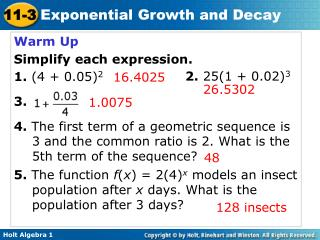Download PresentationWarm Up Simplify each expression. 1. (4 + 0.05) 2 3.

# Warm Up Simplify each expression. 1. (4 + 0.05) 2 3. - PowerPoint PPT PresentationDownload Presentation## Warm Up Simplify each expression. 1. (4 + 0.05) 2 3.

- - - - - - - - - - - - - - - - - - - - - - - - - - - E N D - - - - - - - - - - - - - - - - - - - - - - - - - - -
##### Presentation Transcript

1. Warm Up Simplify each expression. 1. (4 + 0.05)2 3. 4. The first term of a geometric sequence is 3 and the common ratio is 2. What is the 5th term of the sequence? 5. The function f(x) = 2(4)x models an insect population after x days. What is the population after 3 days? 2. 25(1 + 0.02)3 16.4025 26.5302 1.0075 48 128 insects

2. Learning Target Students will be able to: Solve problems involving exponential growth and decay.

3. Exponential growth occurs when an quantity increases by the same rate r in each period t. When this happens, the value of the quantity at any given time can be calculated as a function of the rate and the original amount.

4. The original value of a painting is \$9,000 and the value increases by 7% each year. Write an exponential growth function to model this situation. Then find the painting’s value in 15 years.

5. A sculpture is increasing in value at a rate of 8% per year, and its value in 2000 was \$1200. Write an exponential growth function to model this situation. Then find the sculpture’s value in 2006.

6. A common application of exponential growth is compound interest. Recall that simple interest is earned or paid only on the principal. Compound interest is interest earned or paid on both the principal and previously earned interest.

7. Reading Math • For compound interest • annually means “once per year” (n = 1). • quarterly means “4 times per year” (n =4). • monthly means “12 times per year” (n = 12).

8. Write a compound interest function to model each situation. Then find the balance after the given number of years. \$1200 invested at a rate of 2% compounded quarterly; 3 years. \$15,000 invested at a rate of 4.8% compounded monthly; 2 years.

9. Write a compound interest function to model each situation. Then find the balance after the given number of years. \$1200 invested at a rate of 3.5% compounded quarterly; 4 years \$4000 invested at a rate of 3% compounded monthly; 8 years

10. Exponential decay occurs when a quantity decreases by the same rate r in each time period t. Just like exponential growth, the value of the quantity at any given time can be calculated by using the rate and the original amount.

11. Notice an important difference between exponential growth functions and exponential decay functions. For exponential growth, the value inside the parentheses will be greater than 1 because r is added to 1. For exponential decay, the value inside the parentheses will be less than 1 because r is subtracted from 1.

12. The population of a town is decreasing at a rate of 3% per year. In 2000 there were 1700 people. Write an exponential decay function to model this situation. Then find the population in 2012. The fish population in a local stream is decreasing at a rate of 3% per year. The original population was 48,000. Write an exponential decay function to model this situation. Then find the population after 7 years.

13. A common application of exponential decay is half-life of a substance is the time it takes for one-half of the substance to decay into another substance.

14. Astatine-218 has a half-life of 2 seconds. Find the amount left from a 500 gram sample of astatine-218 after 10 seconds. Bismuth-210 has a half-life of 5 days. Find the amount of bismuth-210 left from a 100-gram sample after 5 weeks. (Hint: Change 5 weeks to days.) HW pp. 10-20,23-33 Odd,35-48,50,52,54,55-60